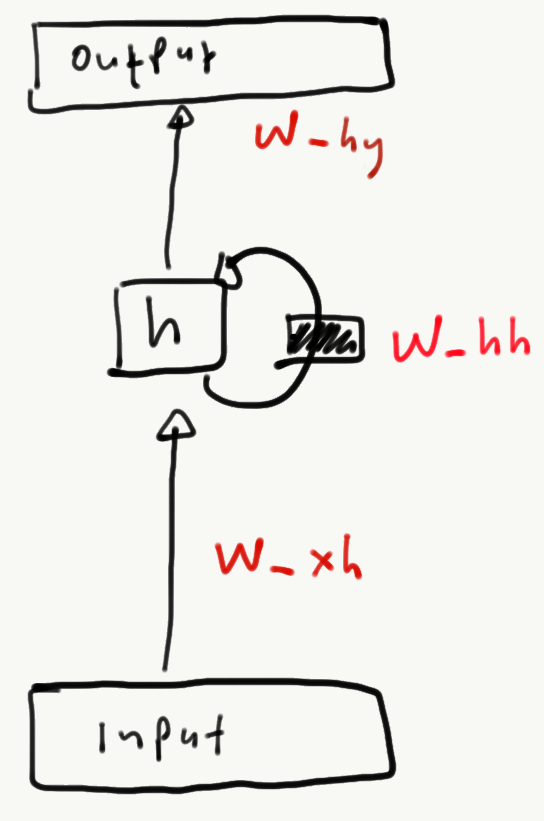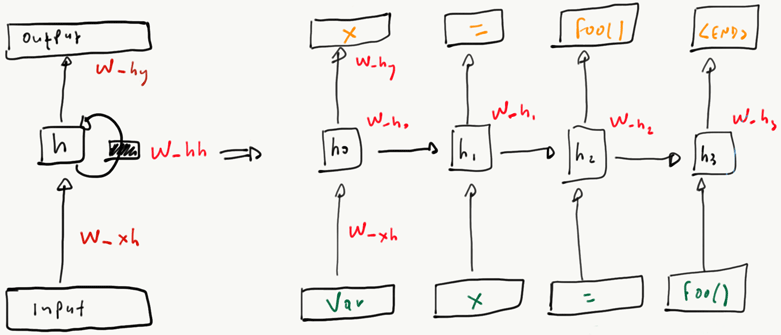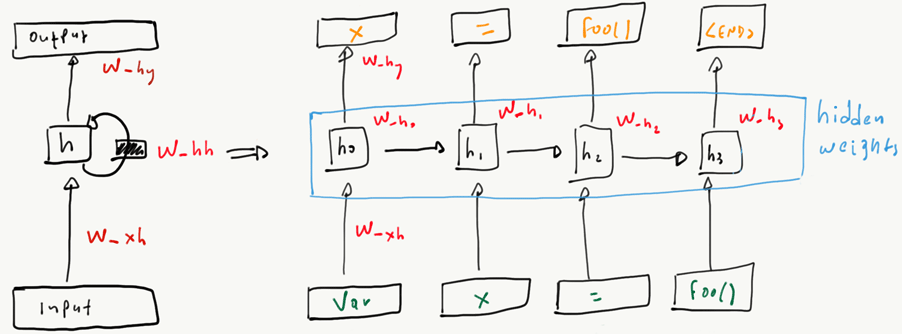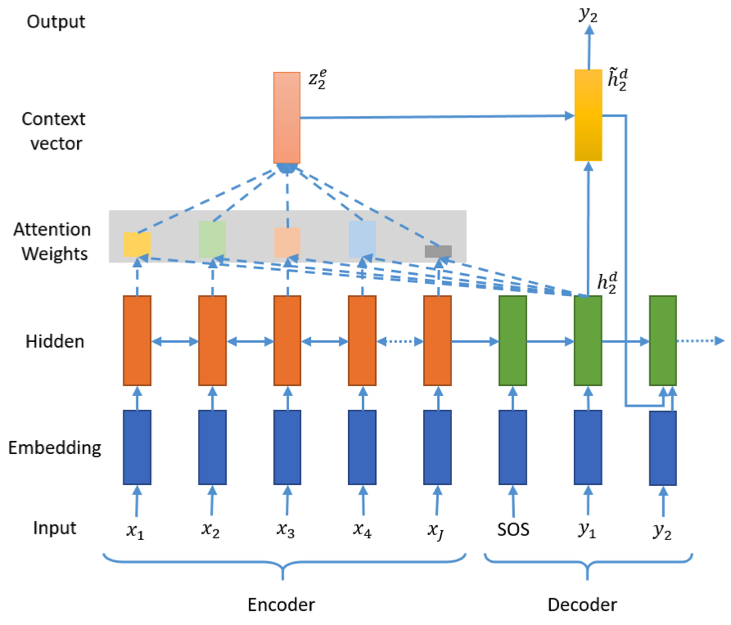# Representing source code

• ML models work on numerical vectors
• Source code comprises textual data
• But also semantics inherited through the PL definition

Source code representation is the task of converting source code data to data that can be consumed by ML models. The task attempts to optimize the loss of information during the conversion.

## Forms of representation

• Text-only representations
• Treating source code as natural language text
• Structured representations
• Using the AST and / or semantic information
• Combined representations

## Tokens

Similar to other NLP applications, textual representations treat source code as a tokens.

def add(x, y):
return x + y

Tokens comprise:

• language syntax: def, (, ), :, +, return
• developer-defined identifiers: add, x, y

Tokens can be considered:

• as a list / sequence (most frequently)
• as a set / bag, when order is not important

## Learning with tokens

NLP approaches use a dictionary $$d: |D| \rightarrow \mathbb{R}$$, to convert textual representations to numerical vectors. A dictionary is built by mapping all individual tokens ($$|D|$$) in a corpus to a unique number in $$\mathbb{R}$$.

Given $$d$$, we can construct a function $$\epsilon: D \rightarrow \mathbb{R}^k$$, where:

• $$D$$ is a sequence / set of tokens representing our input
• $$k$$ is the number of dimensions

that will convert a token to a vector of $$k$$ dimensions. The $$\epsilon$$ function is called the embedding function.

## One-hot encoding

The simplest $$\epsilon$$ uses a vector $$V$$ of size $$|d|$$, i.e., equal to the vocabulary size, to encode tokens.

All elements of $$|V|$$ are 0 except the index position of token $$t$$.

This is called the one-hot encoding.

Q: What is the biggest problem of one-hot encodings?

A: The representation is extremely sparse, so lots of space is wasted.

## Token embeddings

More useful embedding $$\epsilon$$ functions produce vectors of size $$s$$ where $$s << |D|$$.

Useful embedding functions can be learned by:

• Pre-training with external models, such as Word2Vec or FastText
• Training them jointly with the model they serve

D: What are the advantages of each approach?

A: In joint training, the embeddings fit the task perfectly, but cannot be reused for other tasks. Pre-training is task agnostic, so embeddings may be reused (e.g., across tasks).

## Embedding tokens: Word2Vec

Word2Vec builds embeddings by solving a fake problem:

• Assume that $$t_i$$ is the token we are currently processing
• Tokens $$t_{i-2}$$, $$t_{i-1}$$, $$t_{i+1}$$ $$t_{i+2}$$ are its context
• We give the context to a feedforward layer $$V$$ and we ask it to predict $$t_i$$ (CBOW)
• Alternatively, we give $$t_i$$ as input and ask it to predict the context (skip-gram)
• We keep $$V$$ as the embedding of $$t_i$$

## Embedding tokens: FastText

Word2Vec is a closed vocabulary model: Tokens not encountered during training cannot be embedded during prediction.

FastText solves this, by training on ngrams. The idea is that unseen tokens can be represented by combining the learned ngram embeddings.

On a recent study, FastText embeddings were found to better represent developer’s opinions on identifier similarity 

# Documents as vectors

A document is an abstract term we use to represent a sequence / bag of tokens.

In NLP, a document can be a sentence, a paragraph or a document.

In ML4SE, a document is usually a function/method or an expression.

## From token embeddings to document embeddings

Q Given a source code document and an embedding model for its tokens, how can we obtain an embedding for the document?

Is sequence information important?

• N use one of the max, sum, avg functions on the embedded token bag
• Y use a model that learns token sequences

## Naturalness

Software is a form of human communication; software corpora have similar statistical properties to natural language corpora; and these properties can be exploited to build better software engineering tools.
— Hindle et al.

The repetitive nature of code is called naturalness.

The homonymous seminal paper found that source code is more repetitive than natural language text, so we can get good sequence compression.

## Language models

To exploit naturalness in code documents, we can build sequence embeddings using techniques such as:

• n-grams
• Sequence models

For applications where order does not matter, we can also represent documents with TF-IDF / BM25

## n-gram language models

n-gram models attempt to compute the probability $$P(token|history)$$, i.e., the probability a sequence of tokens (history) is followed by token.

Given the document if True: print("🐔", "🥚") and ignoring syntax tokens, we get the following for various $$n$$.

n n-grams
1-gram if, True, print, 🐔, 🥚
bigram if True, True print, print 🐔, 🐔🥚
trigram if True print, True print 🐔, print 🐔🥚

## Sequence modeling

RNNs and their variations (notably LSTMs) are the basic building block for learning from sequences.

RNNs take a sequence of $$n$$ (embedded) tokens and learn and embedding of the sequence

## What does an RNN look like?The basic RNN

## How does an RNN learn?RNN learning from a sequence

We feed the RNN one token at a time and ask it to predict the next token.

## RNN sequence embeddingsRNN hidden state weights

The weights of the hidden states are used as the input sequence embeddings. Those can be fed to downsteam models, such as:

• Feedforward layers, e.g. to predict labels
• Other RNNs, to learn deeper sequence embeddings

## Sequence to Sequence models

A particularly interesting class of models translates between sequences:

• Source code summarization
• Auto completion
• Type prediction
• Language translation
• Code search

## How does seq2seq translation work?

• A (stack of) RNN(s) encodes the input
• The sequence embedding is fed to:
• a (stack of) RNN(s) that predicts the output sequence
• a parallel feed-forward net that learns to weight the sequence embedding cells based on the output (attention)
• The loss is computed by comparing the ground truth with the decoder output
• At prediction time, beam search is employed to learn the most probable sequences given top-n predictions at each step

## How does attention work?Seq2Seq with attention

Image credit: 

# Common problems

The following problems are particular to the application of NLP techniques on source code

• The vocabulary problem
• The long-range dependency problem

## The vocabulary problem

There are only two hard things in Computer Science: cache invalidation and naming things.
Phil Karlton
• C standard library: strcpy
• RabbitMQ: UnexpectedSuccessException
• PyTorch: Transformer
• Spring framework: SimpleBeanFactoryAwareAspectInstanceFactory

While software is more repetitive than natural language, software vocabulary is much more diverse as developers can create new identifiers at will.

## Vocabulary statistics

In 14k (deduplicated ) repositories, Babii et al.  found:

• 11.5M unique tokens
• After removing comments and whitespace: 8M
• After splitting words (FooBar ->Foo, Bar): 975k

It is impossible for NLP models (e.g., ngrams, RNNs) to learn long tail distributions, e.g., tokens appearing 1-2 times in the vocabulary.